# Introduction to Matrices

 A matrix is used to arrange number or data into rows and columns. Here is an example of a matrix: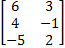Matrices (the plural of matrix) can be classified by listing first the number of rows then the number of columns. The matrix above is a 3x2 matrix because it has 3 rows and 2 columns. The following matrix: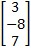is a 3x1 matrix because it has 3 rows and only 1 column. Each number within a matrix is called an entry. Matrices are considered to be equal only if all the corresponding entries are equal. Thus,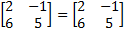but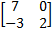does not equal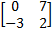because the order has been switched and thus the corresponding entries do not match. Practice: Classify each matrix. 1)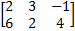2)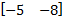3)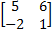4)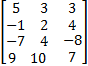5)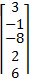Answers: 1)2 x 3 2)1 x 2 3)2 x 2 4)4 x 3 5)5 x 1